### 程序员囧辉：只有知识的积累，而没有深度思考，终究无法完整蜕变。@ TOC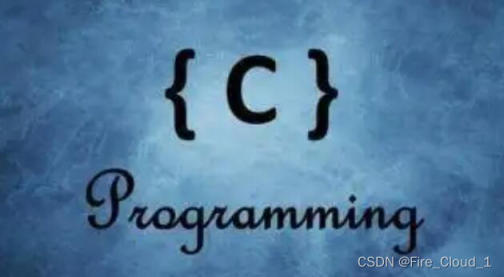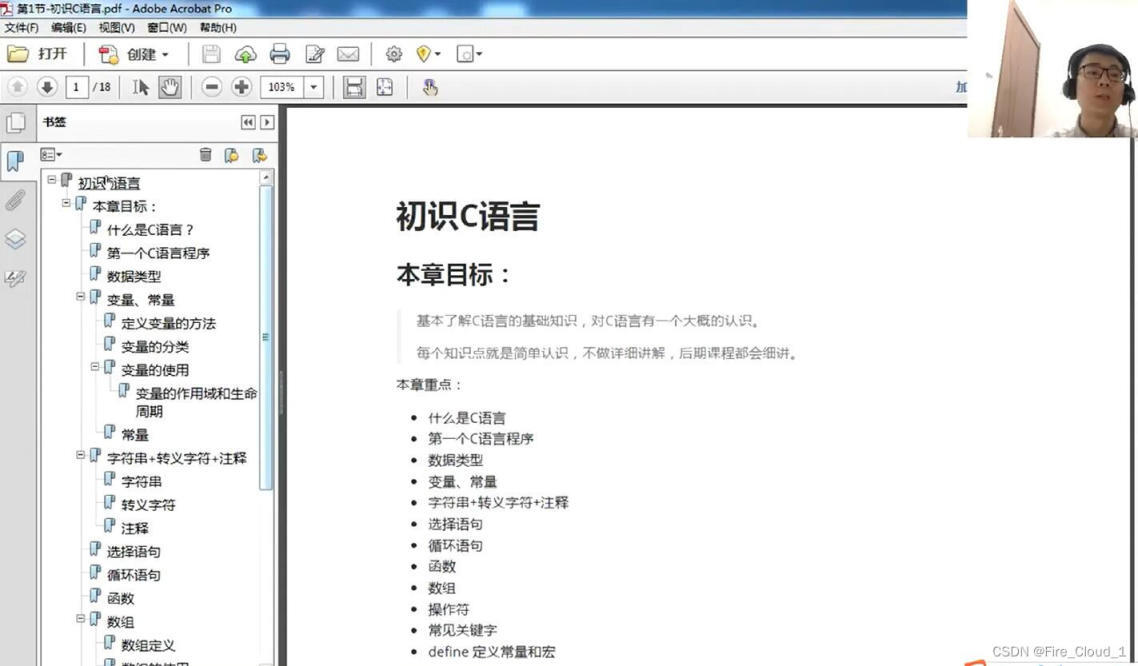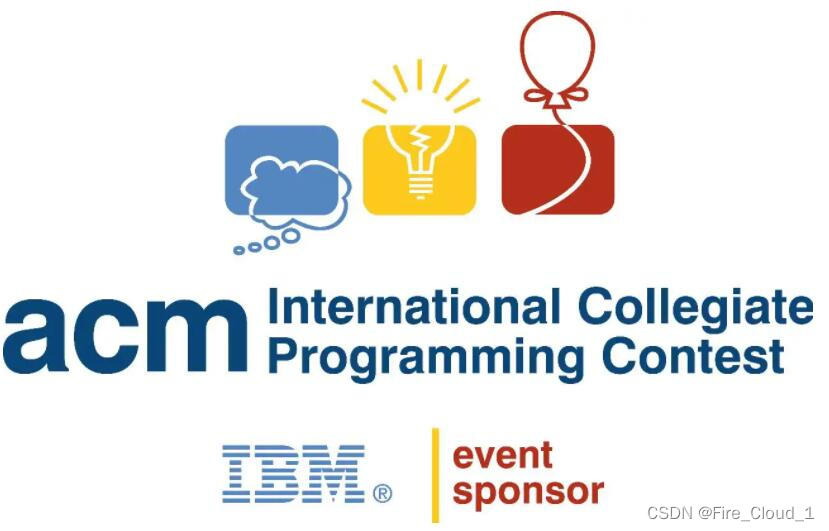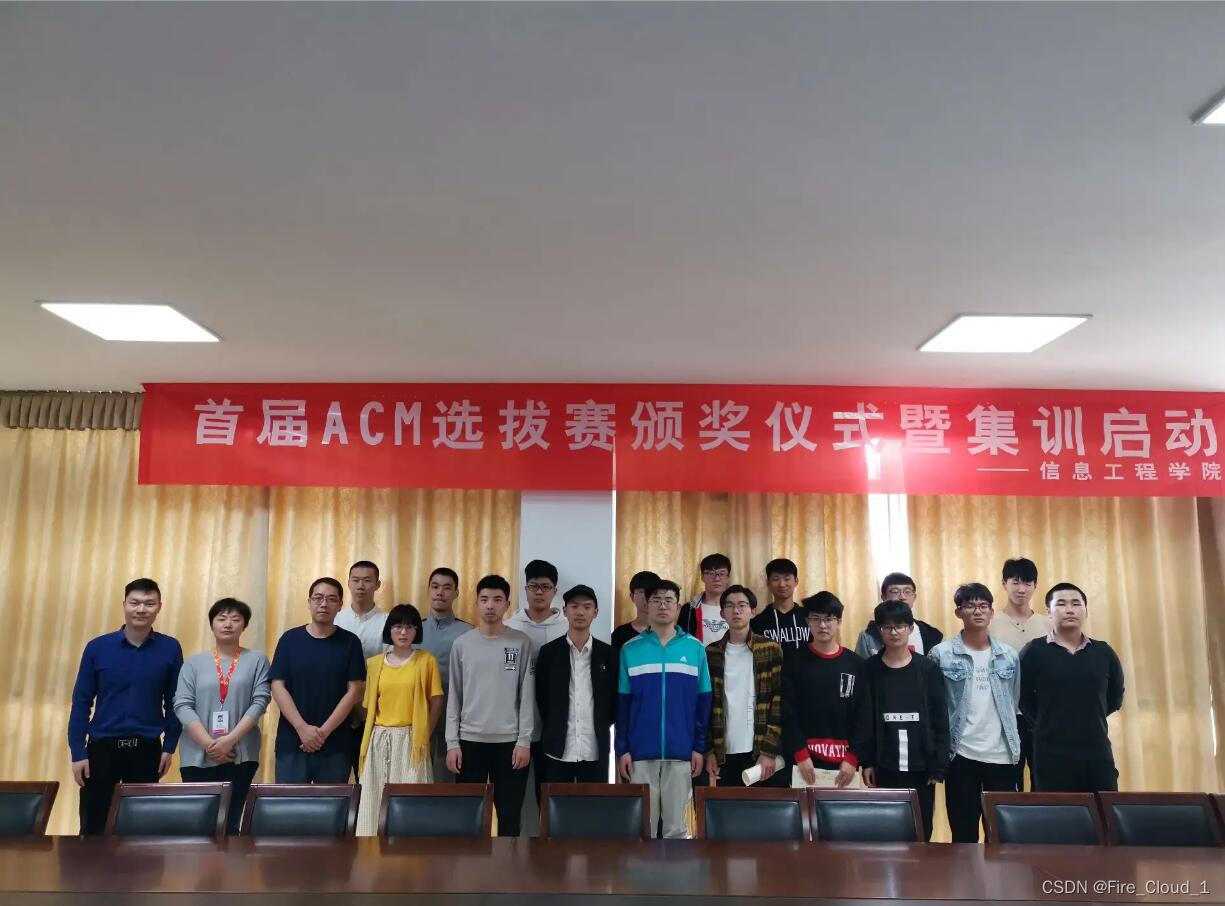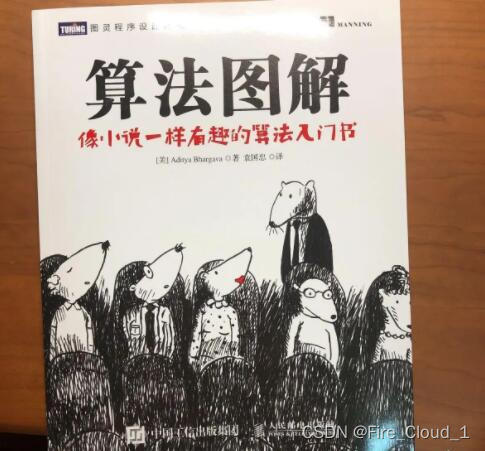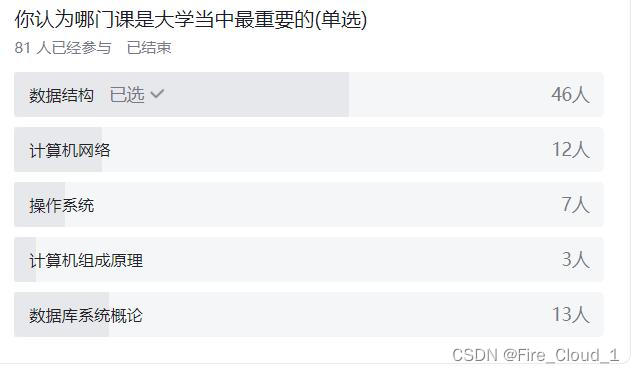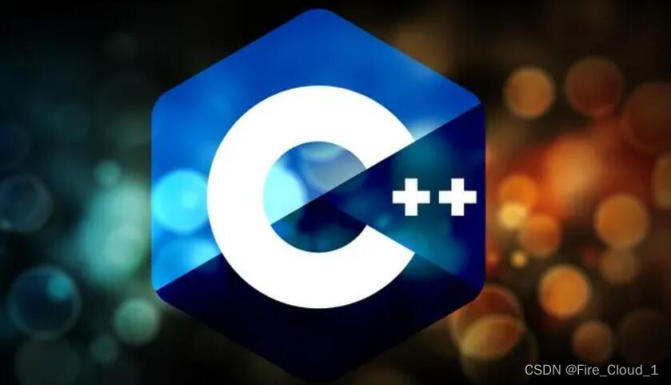C++这门课的话我们是大一第一学期开设的，因为寒假在学习数据结构，所以就没系统地去学 C++ 了，开课前只会 cin 和 cout，C++这们编程语言的话是包含了 C 的全部内容，只是增加一些面向对象的知识点，初次接触面向对象的编程语言，刚开始确实是不太适应，可能因为之前没有接触过，所以没有这个思想，但是在学习了一个多月后，就差不多适应了，类和对象的三大特性是封装、继承和多态，这也是 C++ 区别于 C 的一块部分，而且在 C++ 这一块的指针部分，感觉比 C 更加深入了一些，==指针常量、常量指针，指针数组、数组指针，指针函数、函数指针，除此之外还有对象指针==，幸好这些鹏哥在 C 语言里面也有讲到，所以就又回去看鹏哥的视频了，不得不说，鹏哥在指针这一块讲得太详细，简直让我膜拜，各种底层内存图和堆栈原理图，我也只是学习了它的一些皮毛而已

C++这门语言在我们 ACMer 中，最重要的应该就是 STL 了，这个的话我也有写过相应的博客，六大组件，容器、算法、迭代器，都是我们需要掌握的知识点，STL 在实际的做题过程中还是用的比较多的，像 vector 容器、deque 容器、pirority_queue 优先队列。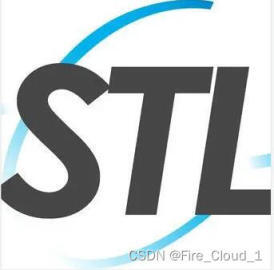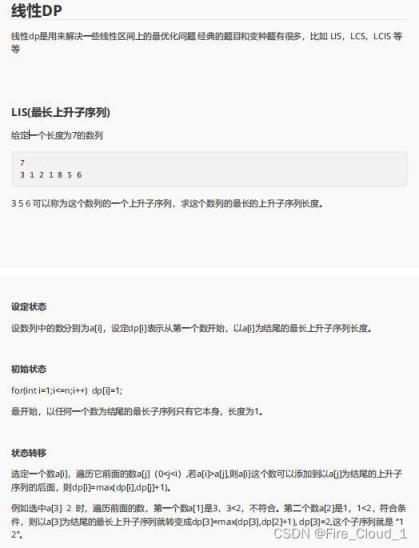ing namespace std; int a,dp,ans,n; int main (){ cin>>n; for(int i=1;i<=n;i++){ cin>>a[i]; dp[i]=1;//初始状态 } ans=1; for(int i=1;i<=n;i++){ for(int j=1;j<=i;j++){ if(a[j]> threeSum (vector& nums) { vector> result; sort (nums.begin (),nums.end ()); //返回不是下标，因而可以排序 //a + b + c = 0 //nums[i] = a nums[left] = b nums[right] = c for(int i = 0;i < nums.size (); ++i) { if(nums[i] > 0) //因为是排过序的数组，前面一定是最小的，如果 a > 0，则相加不可能为0 return result; //对a去重 //要考虑到[-1,-1,2]，若不写 i > 0，则会略过这一种情况 // if (nums[i] == nums[i - 1]) continue; if(i > 0 && nums[i] == nums[i - 1]) continue; int left = i + 1; //左指针为 i + 1 int right = nums.size () - 1; //右指针为 nums.size () - 1 while(left < right) { //left 和 right 不能相等，因为所求集合为三元组，若 left == right，则指向统一元素 //此处不可对左右指针去重,否则会漏掉[0,0,0]这一种情况 // if (left < right && nums[left] == nums[left + 1]) left++; // if (left < right && nums[right] == nums[right - 1]) right--; //因排过序，为升序 if(nums[i] + nums[left] + nums[right] < 0) left++; //左指针后移，取得更大数字 else if(nums[i] + nums[left] + nums[right] > 0) right--; //右指针前移，取得更小数字 else{ //表示刚好取到一组之和为 0 的三个数 result.push_back (vector{nums[i],nums[left],nums[right]}); //在获取一个三元组后，前后指针继续移动去重b,c //需使用 while，直到符合正确条件为止，因为b,c可能连着相等 while(left < right && nums[left] == nums[left + 1]) left++; while(left < right && nums[right] == nums[right - 1]) right--; //找到正确答案后，双指针同时收缩 left++; right--; } } } return result; //返回最后的可能结果 } };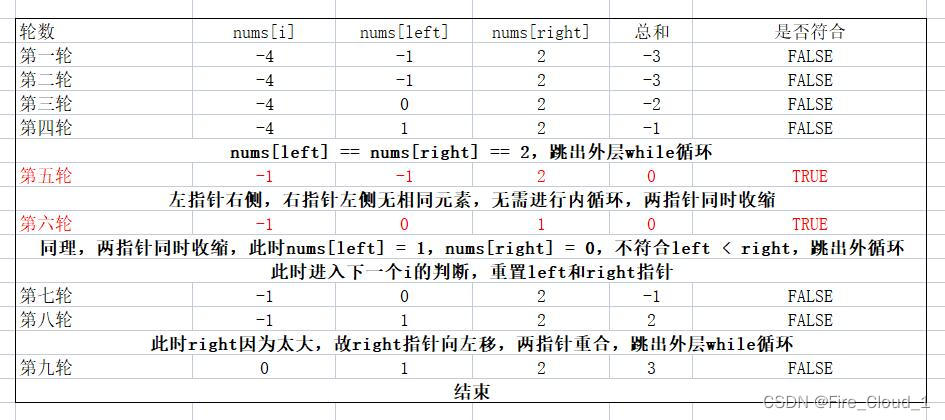## 人生间的心路历程

"学而不思则罔，思而不学则殆"

“只有知识的积累，而没有深度思考，终究无法完整蜕变。”——《感动中国》年度热心观众，程序员囧辉